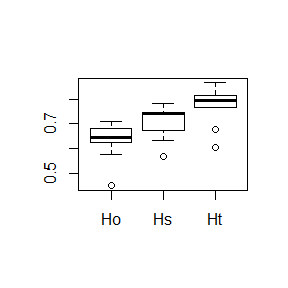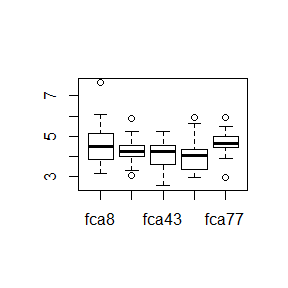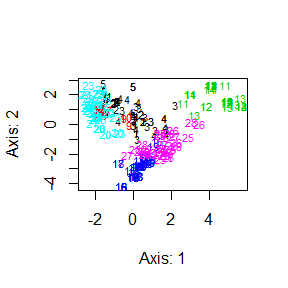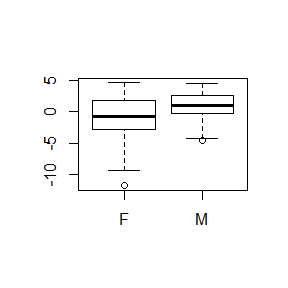# Introduction

This vignette documents the latest developments in hierfstat. Refer to the other vignettes for an introduction to the package

hierfstat can now read genindobjects (from package adegenet). Note however that only some genetic data types will be properly converted and used. The alleles need to be encoded either as integer (up to three digits per allele), or as nucleotides (c("a","c","g","t","A","C","G","T")).

library(adegenet)
library(hierfstat)
data(nancycats)
is.genind(nancycats)
##  TRUE
boxplot(basic.stats(nancycats)$perloc[,1:3]) # boxplot of Ho, Hs, Ht# Descriptive statistics The function you are the most likely to want using is basic.stats (it calculates $$H_O$$, $$H_S$$, $$F_{IS}$$, $$F_{ST}$$ etc…). You can also get e.g. allele.count and allelic.richness, a rarefied measure of the number of alleles at each locus and in each population. For instance, below is a boxplot of the allelic richness for the 5 first loci in the nancycats dataset boxplot(t(allelic.richness(nancycats)$Ar[1:5,])) #5 first loci# Populations

Population statistics are obtained through basic.stats or wc (varcomp.glob can also be used and will give the same result as wc for a one level hierarchy). For instance, $$F_{IS}$$ and $$F_{ST}$$ in the Galba truncatula dataset provided with hierfstat are obtained as:

data(gtrunchier)
wc(gtrunchier[,-2])
## $FST ##  0.540894 ## ##$FIS
##  0.8154694
varcomp.glob(data.frame(gtrunchier[,1]),gtrunchier[,-c(1:2)])$F #same ## gtrunchier...1. Ind ## Total 0.540894 0.9152809 ## gtrunchier...1. 0.000000 0.8154694 Confidence intervals on these statistics can be obtained via boot.vc: boot.vc(gtrunchier[,1],gtrunchier[,-c(1:2)])$ci
##       H-Total F-Pop/Total F-Ind/Total  H-Pop F-Ind/Pop   Hobs
## 2.5%   0.6556      0.4856      0.9047 0.2670    0.7677 0.0557
## 50%    0.7456      0.5401      0.9147 0.3413    0.8147 0.0631
## 97.5%  0.8163      0.6200      0.9259 0.4121    0.8470 0.0699

boot.ppfisand boot.ppfst provide bootsrap confidence intervals (bootstrapping over loci) for population specific $$F_{IS}$$ and pairwise $$F_{ST}$$ respectively.

# Genetic distances

genet.dist estimates one of 10 different genetic distances between populations as described mostly in Takezaki & Nei (1996)

(Ds<-genet.dist(gtrunchier[,-2],method="Ds")) # Nei's standard genetic distances
##           1         2         3         4         5
## 2 0.4272210
## 3 1.1402899 1.8235430
## 4 0.8387367 0.9540338 1.6638485
## 5 0.6967425 0.6205417 2.5798363 0.8767008
## 6 0.9411656 0.9742812 1.1553423 0.5243353 1.1911894

# Population Principal coordinate analysis

Principal coordinate analysis can be carried out on this genetic distances:

pcoa(as.matrix(Ds))

# Individual PCA

hierfstat has a function indpca carrying out Principal component analysis on individual genotypes

x<-indpca(gtrunchier[,-2],ind.labels=gtrunchier[,2])
plot(x,col=gtrunchier[,1],cex=0.7)# Sex-biased dispersal

A new function to detect sex-biased dispersal, sexbias.test based on Goudet et al. (2002) has been implemented. To illustrate its use, load the Crocidura russula data set. It consists of the genotypes and sexes of 140 shrews studied by Favre et al. (1997). In this species, mark -recapture showed an excess of dispersal from females, an unusual pattern in mammals. This is confirmed using genetic data:

data("crocrussula")
aic<-AIc(crocrussula$genot) boxplot(aic~crocrussula$sex)tapply(aic,crocrussula$sex,mean) ## F M ## -1.1602396 0.9770438 sexbias.test(crocrussula$genot,crocrussula$sex) ##$call
## sexbias.test(dat = crocrussula$genot, sex = crocrussula$sex)
##
## $statistic ## t ## -4.117605 ## ##$p.value
##  8.097862e-05

# Simulating genetic data

It is now possible to simulate genetic data from an island model, either at equilibrium via sim.genot or for a given number of generations via sim.genot.t. These two functions have several arguments, allowing to look at the effect of population sizes, inbreeding, migration and mutation. the number of independant loci and number of alleles per loci can also be specified.

# Exporting to other programs

hierfstatcan now export to files suitable for Bayescan, plink and structure. Functions to import from these program can be found in adegenet. The functions to export to these programs are write.bayescan, write.ped and write.structrespectively.